# Catch up on the latest AI articles# DualNet, A New Framework For Two Sub-network Coordination

3 main points
✔️ Two parallel DCNNs are proposed to work together to form a DualNet that learns complementary features from each other
✔️ A corresponding learning strategy consisting of iterative learning and joint fine-tuning is also proposed to ensure that the two sub-networks cooperate well.
✔️ Experimental evaluation of DualNet based on CaffeNet, VGGNet, NIN, and ResNet on CIFAR-100, Stanford Dogs, and UEC FOOD-100, all achieving higher accuracy than the baseline

DualNet: Learn Complementary Features for Image Recognition
written by Saihui HouXu LiuZilei Wang
Published in: 2017 IEEE International Conference on Computer Vision (ICCV)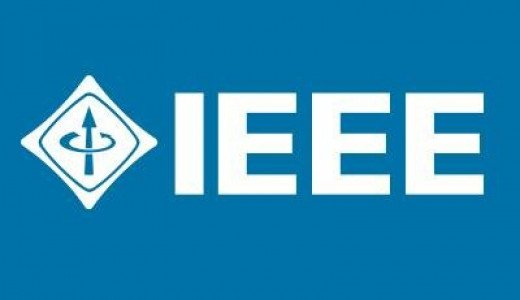The images used in this article are from the paper, the introductory slides, or created based on them.

## first of all

In recent years, there has been a lot of research on deep convolutional neural networks (DCNNs), which have significantly improved the performance of various visual tasks. The success of DCNNs has been attributed largely to the depth of the network architecture and the end-to-end learning approach that can learn a hierarchical representation of the input.

As such, networks are generally designed to be deeper or wider to make DCNNs perform better.

In this paper, a framework, DualNet, is proposed to efficiently learn more accurate representations in image recognition, as shown in Fig. 1.
DualNet links two parallel DCNNs to learn features that are complementary to each other, The objective is to extract richer features from images by linking two parallel DCNNs and learning complementary features.
Specifically, a double-width network is constructed by arrangingExtractor, an end-to-end DCNN consisting of two logical parts, Extractor (feature extractor) and Classifier (image classifier ), which is used as an extractor in DualNet.

By doing so, two streams of features can be extracted for the input image, which is then aggregated to form a unified representation and passed to the Fused Classifier for the overall classification.
On the other
hand, two Auxiliary Classifiers are added behind the extractor of each sub-network to allow separately learned features to be discriminated independently, and by weighting these three Classifiers, complementary constraints are imposed This is the key point of DualNet.

The paper also proposes a new framework, "DualNet", as well as a corresponding learning strategy consisting of iterative learning and joint fine-tuning so that the two sub-networks cooperate well.

Compared with the method of simply doubling the layer width, this method is practical without much memory cost and can bring significant improvement in image recognition.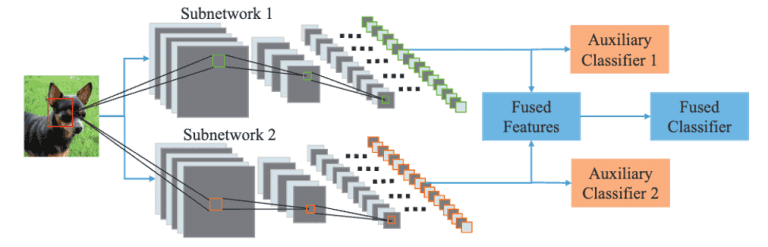Figure 1: DualNet Overview

## proposed method

As explained in the previous section, we can say that DualNet is characterized by linking two networks and learning complementary features from input images.
By learning details of the object of interest in one network that is missing in the other, after fusion, a richer and more accurate image representation can be extracted for recognition.

In particular, in designing DualNet, we follow the following principles.

• The features after fusion are the most discriminating compared to the features extracted in each sub-network
• Must be a generic framework that works well on typical DCNNs such as VGGNet and ResNet, and common datasets such as CIFAR-100
• From a computational cost perspective, the network should be as efficient as possible for training and testing without reducing the mini-batch size and should support Tesla K40 GPUs (12 GB memory limit)
• To ensure generalization capability and computational efficiency, only simple fusion methods such asSUM, MAX, and Concat are considered, emphasizing the coordination and complementarity of the two subnets

The following sections describe in detail each of the DualNet architectures and the corresponding learning methods.

## DualNet

DualNetperformscomplementary learning by using two models as a subnetwork and any existing model can be used.

An example architecture of DualNet From CaffeNet (DNC) is shown in Figure 2.
For simplicity, we denote the two CaffeNets as S1 and S2.
In particular, the end-to-end CaffeNet is logically partitioned into two functional parts, Extractor and Classifier.
The partitioning of Extractor and Classifier is not a fixed one and theoretically can be done at any layer, but here it is done in pool5 (The partitioning is done in pool5. (There were some reasons why we chose pool5 for the partitioning, but we omit them here. If you are interested, please check the paper.)

Overall, DNC has a symmetric architecture where S1Extractor and S2Extractor are placed in parallel and the feature maps generated by them are integrated into the Fused Classifier.
We also add auxiliary S1Classifier and S2Classifier to allow the features generated by each feature extractor to be identified independently.
We then impose complementary constraints by weighting the three classifiers.

SUM was chosen as the fusion method because of its simplicity and because it inherits the parameters of the last fully connected layer (classifier) of the original CaffeNet, where the coefficients are fixed to {0.5,0.5}.

The same method was applied to 16-layer VGGNet, NIN, and ResNet to constructDualNet From VGGNet (DNV), DualNet From NIN (DNI) andDualNet From ResNet (DNR), respectively.

In DNV, S1 Extractor and S2 Extractor consist of layers before pool5 of VGGNet.
Since NIN and ResNet do not have a fully connected layer and the input size is as small as 32×32, the last convolutional layer (e.g., cccp5 in NIN) is used to average the two sub The feature maps of the two subnetworks are averaged together in the last convolutional layer (e.g., cccp5 in NIN) and the last convolutional layer is used as prediction 3.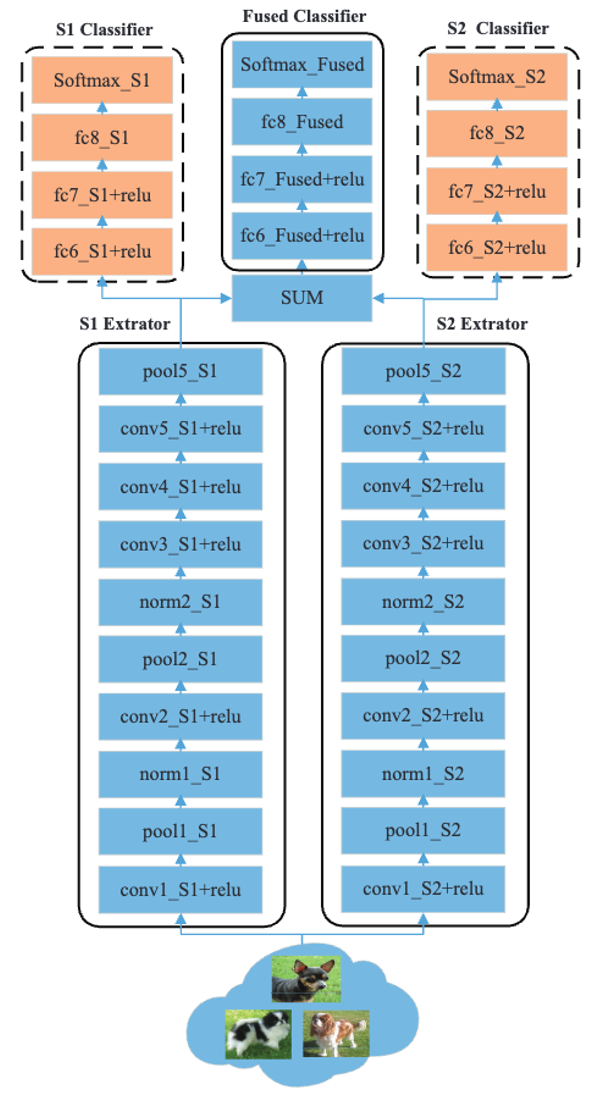Figure 2: DualNet From CaffeNet (DNC) Architecture Example

## How to learn the DNC

The learning process of DNC consists of two major parts: Iterative Training and Joint Finetuning, as shown in Fig. 3.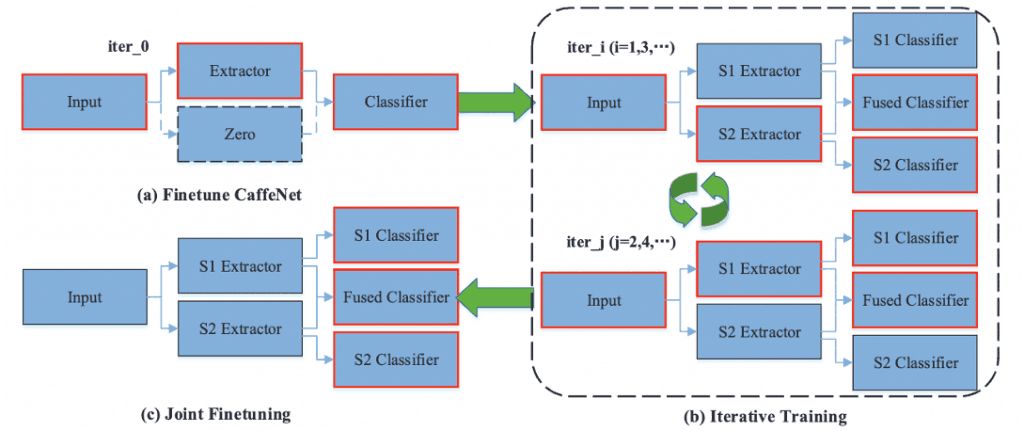Figure 3: How DNC is learned

### learning by rote

Iterative learning is an iterative process between S1 Extractor and S2 Extractor where one is fixed and the other is fine-tuned.
This method allows one extractor to explicitly learn complementary features to the other at each iteration, resulting in more discriminative fusion features.

We will explain the concrete method of iterative learning by taking DNC as an example.
First, the Extractor and Fused Classifier of one of the two sub-networks (here, S1 for simplicity) is trained (iter_0).
Here, the S1 Auxiliary Classifier is not trained because it is omitted.

The parameters obtained by training are used as the initial values of the S1 Extractor and Fused Classifier, and iterative training is performed from iter_1 onwards.

(iter_i, i=1,3,...) Now, we will train S2 Extractor, S2 Classifier, and Fused Classifier.
During training, the parameters of the S1 Extractor are fixed and are not updated.

Specifically, each module includingS2 Extractor, S2 Classifier, and Fused Classifier is optimized according to the loss function defined as follows

$L_1=L_{\text {Fused }}+\lambda_{S2} L_{S2}$

where $L_{\text {Fused }}$ and $L_{S2}$ are the cross-entropy losses computed by Softmax Fused and SoftmaxS2, and the loss weight $\lambda_{S2}$ is 0.3.
The second term is for regularization of the learning and $\lambda_{S2}<1$ is to inform the S2 Extractor that the Fused Classifier is more important in the optimization.

Also,(iter_j, j=2,4,... ), the S1 Extractor, S1 Classifier, and Fused Classifier are trained.
During training, the parameters of the S2 Extractor are fixed and are not updated.
Specifically, each module includingS1 Extractor, S 1 Classifier, and Fused Classifier is optimized according to the loss function defined as follows

$L_2=L_{\text {Fused }}+\lambda_{S 1} L_{S 1}$

where $L_{\text {Fused }}$ and $L_{S1}$ are the cross-entropy losses computed by Softmax Fused and SoftmaxS1, and the loss weight $\lambda_{S1}$ is 0.3.
The second term is for regularization of the learning, and $\lambda_{S1}<1$ is to inform the S1 Extractor that the Fused Classifier is more important in the optimization.

The DualNet thus trained is evaluated by comparing the output of the Fused Classifier with a base model such as CaffeNet.
It is also possible to calculate the probability of each class by combining the predictions of the three classifiers.

$\textit{score}=\textit{score}_{\text {Fused }}+\lambda_{S 2}\textit{score}_{S 2}+\lambda_{S 1}\textit{score}_{S 1}$

Here,$\textit{score}_{\text {Fused}}$ and score S2, score S1 means the output of Fused Classifier, S2 Classifier, and S1 Classifier at the test, and these scores are used to evaluate recognition.

### joint fine-tuning

Although there are three classifier modules in DualNet, S1 classifier, S2 classifier, and Fused classifier, their abilities are not fully exploited by iterative learning alone.
Therefore, in this paper, another integration method is proposed to further improve the performance of DualNet.

Since fine-tuning an entire network like DNV is time-consuming and requires large GPU memory, we instead jointly fine-tune the last fully connected layer of the three classifier modules (e.g., fc8 for DNC, ccp6 for DNI) with the following loss function.

$L_3=L_{\text {Fused }}+\lambda_{S 2} L_{S 2}+\lambda_{S 1} L_{S 1}$

where $L_{\text {Fused }}$,$L_{S1}$,$L_{S2}$ are the cross-entropy output by the Fused Classifier, S2 Classifier and S1 Classifier respectivelyand the loss weights $\lambda_{S1}$,$\ lambda_{S2}$ is set to 0.3.

## experimental setup

We evaluate the performance of DualNet From CaffeNet (DNC), DualNet From VGGNet (DNV), DualNetFrom NIN (DNI), and DualNet From ResNet (DNR) on several datasets.

The hyperparameters for iterative learning are the same as for fine-tuning a standard deep model on a particular dataset, with joint fine-tuning reducing the base learning rate by a factor of 10 for a few additional iterations.

## Results and Discussion

The results of comparing the accuracy of standard NIN and ResNet with that of the proposed methods, DualNet From NIN (DNI) andDualNet From ResNet (DNR), for CIFAR100 image classification are shown in Figure 4.

The table in Figure 4 shows the following results from the top respectively.
Here, the right and left differences indicate the differences with and without data expansion applied.

• Results of CIFAR100 image classification using NIN and ResNet
• The results of CIFAR100 image classification using only the output of the Fused Classifier learned by iterative learning.
• Results of CIFAR100 image classification using the weighted combined outputs of the three classifiers trained using iterative learning.
• Results of CIFAR100 image classification using the weighted combined outputs of the three classifiers trained using iterative training plus joint fine-tuning.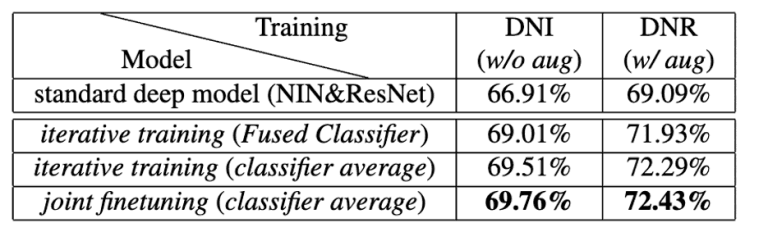Figure 4: Evaluation result 1 of the proposed method(CIFAR-100)

Similarly, for CIFAR100 image classification, the accuracy of the existing methods is compared with the proposed methods, DualNet From NIN (DNI) andDualNet From ResNet (DNR), and the results are shown in Figure 5.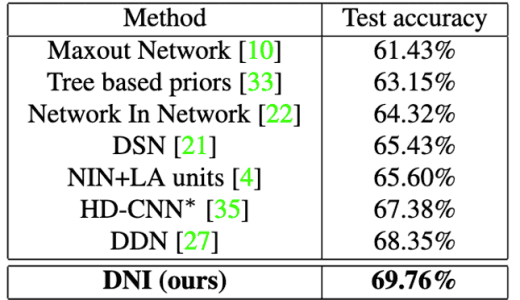Figure 5: Evaluation result 2 of the proposed method (CIFAR-100)

In both results, it can be confirmed that the proposed method shows the best accuracy.
In result 1, the best accuracy was obtained by using joint fine-tuning in addition to iterative learning.

Then, the results of comparing the accuracy of standard CaffeNet and VGGNetwith that of the proposed method, DualNet From CaffeNet (DNC), for the image classification of Stanford Dogs and UEC FOOD-100 are shown in Figure 5.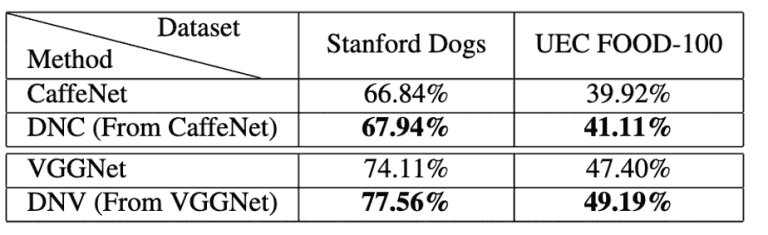Figure 6: Evaluation result 3 of the proposed method (Stanford Dogs, UEC FOOD-100)

For both datasets, the proposed methods, DNC and DNV, perform well and achieve higher accuracy than CaffeNet and VGGNet.

## summary

In this paper, we proposed a general-purpose framework called DualNet for image recognition tasks.
In this framework, two parallel DCNNs cooperatively learn complementary features to build a wider network and obtain a more discriminative representation.

A corresponding learning strategy consisting of iterative learning and joint fine-tuning is also proposed to make the best use of this new framework, "DualNet", so that the two sub-networks cooperate well.

In our experiments, we experimentally evaluated DualNet based on CaffeNet, VGGNet, NIN, and ResNet on CIFAR-100, Stanford Dogs, and UEC FOOD-100 and found that they all achieve higher accuracy than the baseline.
In particular, the accuracy of CIFAR-100 was the best compared to previous studies.

If you have any suggestions for improvement of the content of the article,